Study Materials

# NCERT Solutions for Class 9th Mathematics

Page 4 of 9

## Chapter 13. Surface Areas and Volumes

### Exercise 13.4

Exercise 13.4

Assume π = 22/7 , unless stated otherwise.

Q1. Find the surface area of a sphere of radius:

(i) 10.5 cm                  (ii) 5.6 cm                (iii) 14 cm

Solution: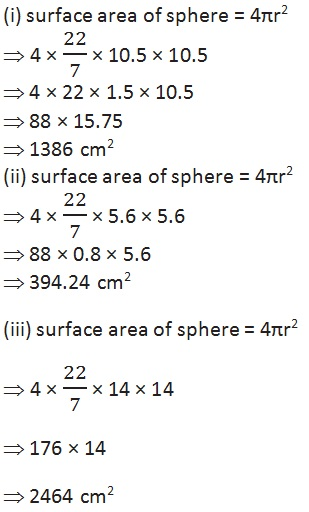Q2. Find the surface area of a sphere of diameter:

(i) 14 cm             (ii) 21 cm                 (iii) 3.5 m

Solution:

(i) Surface area of sphere = 4πr2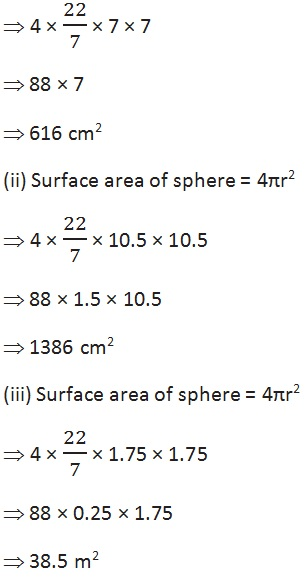Q3. Find the total surface area of a hemisphere of radius 10 cm. (Use π = 3.14)

Solution:

Total surface area of hemisphere = 3πr2

⇒ 3 × 3.14 × 10 × 10

⇒ 3 × 314

⇒ 942 cm2

Q4. The radius of a spherical balloon increases from 7 cm to 14 cm as air is being pumped into it. Find the ratio of surface areas of the balloon in the two cases.

Solution:

Surface area of sphere = 4πr2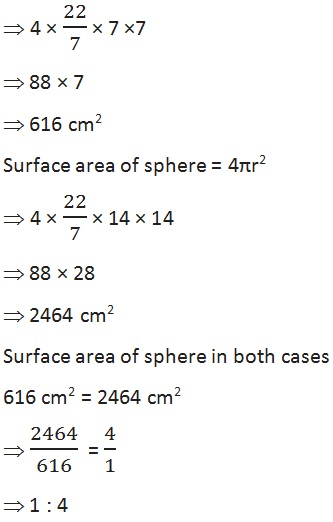Q5. A hemispherical bowl made of brass has inner diameter 10.5 cm. Find the cost of tin-plating it on the inside at the rate of 16 per 100 cm2.

Solution:

Curved surface area of hemisphere = 2πr2Q6. Find the radius of a sphere whose surface area is 154 cm2.

Solution:

Area = 154 cm2

Surface area of sphere = 4πr2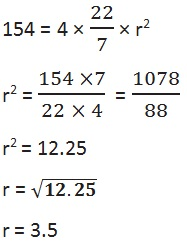Q7. The diameter of the moon is approximately one fourth of the diameter of the earth. Find the ratio of their surface areas.

Solution:

Let the diameter of earth = x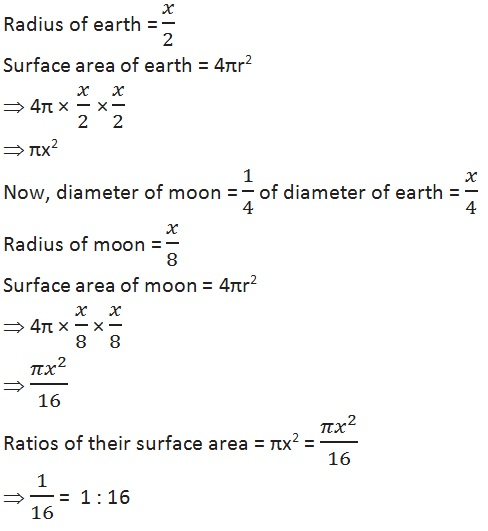Q8. A hemispherical bowl is made of steel, 0.25 cm thick. The inner radius of the bowl is 5 cm. Find the outer curved surface area of the bowl.

Solution:

Inner radius = 5 cm,    width = 0.25 cm,   radius = 5.25 cm

Curved surface area of hemisphere = 2πr2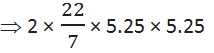⇒ 44 × 0.75 × 5.25

⇒ 173.25

Q9. A right circular cylinder just encloses a sphere of(i) surface area of the sphere,

(ii) curved surface area of the cylinder,

(iii) ratio of the areas obtained in (i) and (ii).

Solution:

Radius of sphere = r,   radius of cylinder = r + r = 2r

(i) surface area of sphere = 4πr2

(ii) curved surface area of cylinder = 2πrh

⇒ 2πr(2r)

4πr2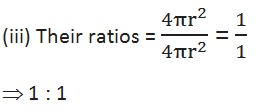Page 4 of 9

Chapter Contents: